## Revision Notes of Chapter 10 Circles Class 9th Math

Topics in the Chapter
• Terms related to Circles
• Fundamentals of Circles
• Theorems
Terms Related to Circles
• The locus of a point which moves in a plane in such a manner that its distance from a given fixed point is always constant, is called a circle.
• The fixed point is called the centre and constant distance is called the radius of the circle.
In the figure, ‘O’ is centre and OP = r is a radius. We denote it by C(O, r).• A line segment, terminating (or having its end points) on the circle is called a chord. A chord, passing through the centre is called a diameter of the circle.
• A line which intersects a circle in two distinct points is called a secant of the circle.
• A line intersecting the circle in exactly one point is called a tangent to the circle.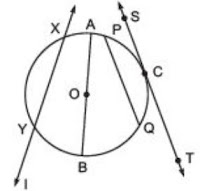• In the figure, PQ is a chord, AB is a diameter, XY is a secant and ST is a tangent to the circle at C.
Note:
(i) Diameter is the longest chord in a circle.
(ii) Diameter = 2 × Radius
• The length of the complete circle is called its circumference, whereas a piece of a circle between two points is called an arc.Note:
(i) A diameter of a circle divides it into two equal arcs, each of which is called a semicircle.
(ii) If the length of an arc is less than the semicircle, then it is a minor arc, otherwise, it is a major arc.

• The region consisting of all points lying on the circumference of a circle and inside it is called the interior of the circle.
• The region consisting of all points lying outside a circle is called the exterior of the circle.
• The region consisting of all points which are either on the circle or lie inside the circle is called the circular region.• A chord of a circle divides it into two parts. Each part is called a segment
• The part containing the minor arc is called the minor segment, and the part containing the major arc is called the major segment.• A quadrilateral of which all the four vertices lie on a circle is called a cyclic quadrilateral. The four vertices A, B, C and D are said to be concyclic points.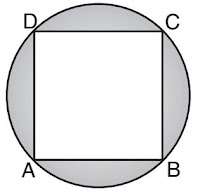Fundamentals of Circles
• Equal chords of a circle (or of congruent circles) subtend equal angles at the centre.
• If two chords of a circle subtend equal angles at the centre, then the chords are equal.
• The line drawn through the centre of a circle to bisect a chord is perpendicular to the chord.
• The perpendicular from the centre of a circle to a chord bisects the chord.
• Equal chords of a circle are equidistant from the centre whereas the equidistant chords from the centre are equal.
• Chords corresponding to equal arcs are equal.
• Congruent arcs of a circle subtend equal angles at the centre.
• The angle subtended by an arc at the centre is double the angle subtended by it at any point on the remaining part.
• Angles in the same segment are equal, whereas the angle in a semicircle is a right angle.
• The sum of either pair of opposite angles of a cyclic quadrilateral is 180º.
• If the opposite angles of a quadrilateral are supplementary, then the quadrilateral is cyclic.
• If a line segment joining two points subtends equal angles at two other points lying on the same side of the line containing the line segment, then the four points are cyclic.
Theorems

Theorem 1

Statement: Equal chords of a circle subtend equal angles at the centre.
Given: AB and CD are chords of a circle with centre O, such that AB = CD.
To prove: ∠AOB = ∠COD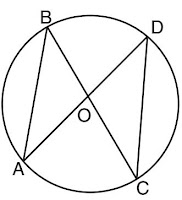Proof:
In △AOB and △COD,
AO = CO (radii of the same circle)
BO = DO (radii of the same circle)
AB = CD (given)
∴ △AOB ≅ △COD (SSS)
Hence, ∠AOB = ∠COD (c.p.c.t.)

Theorem 2

Statement: If the angles subtended by the chords of a circle at the centre are equal, then the chords are  equal.
Given: Two chords PQ and RS of a circle C(O, r), such that ∠POQ = ∠ROS.
To prove: PQ = RS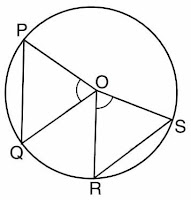Proof: In △POQ and △ROS,
OP = OQ = OR = OS = r (radii of the same circle)
and ∠POQ = ∠ROS (given)
∴ △POQ ≅ △ROS (SAS)
∴ PQ = RS. (c.p.c.t.)

Theorem 3

Statement: The perpendicular from the centre of a circle to a chord bisects the chord.
Given: AB is the chord of a circle with centre O and OD ⊥ AB.
To prove: AD = DB  Construction: Join OA and OB.Proof:
In △ODA and △ODB,
OA = OB (radii of the same circle)
OD = OD (common)
∠ODA = ∠ODB (each is a rt. angle)
△ODA ≅ △ODB (R.H.S.)

Theorem 4

Statement: The line drawn through the centre of a circle to bisect a chord is perpendicular to the chord.
Given: A chord PQ of a circle C(O, r) and L is the mid-point of PQ.
To prove: OL ⊥ PQ.  Construction: Joint OP and OQ.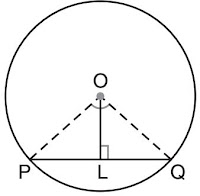Proof:
In △OLP and △OLQ,
OP = OQ (radii of the same circle)
PL = QL (given)
OL = OL (common)
∴ △OLP ≅ △OLQ (SSS)
Also, ∠OLP + ∠OLQ = 180° (linear pair)
∴ ∠OLP = ∠OLQ = 90°
Hence, OL ⊥PQ

Theorem 5

Statement: There is one and only one circle passing through three given non-collinear points.

Theorem 6

Statement: Equal chords of a circle (or of congruent circles) are equidistant from the centre (or centres).
Given: AB and CD are two equal chords of a circle.  OM and ON are perpendiculars from the centre to the chords AB and CD.
To prove: OM = ON.
Construction: Join OA and OC.Proof:
In △AOM and △CON,
OA = OC (radii of the same circle)
MA = CN (Since, OM and ON are perpendicular to  the chords and it bisects the chord and AM = MB, CN = ND)
∠OMA = ∠ONC = 90°
∴ △AOM ≅ △CON (R.H.S.)
∴ OM = ON (c.p.c.t.)
Equal chords of a circle are equidistant from the centre.

Theorem 7

Statement: Chords equidistant from the centre of a circle are equal in length.
Given: OM and ON are perpendiculars from the centre to the chords AB and CD and OM = ON.
To prove: Chord AB = Chord CD.
Construction: Join OA and OC.Proof:
OM ⊥ AB ⇒ 1/2 AB = AM
ON ⊥ CD ⇒ 1/2 CD = CN
Consider △AOM and △CON,
OA = OC (radii of the same circle)
OM = ON (given)
∠OMA = ∠ONC = 90° (given)
△AOM ≅ △CON (RHS congruency)
AM = CN ⇒ 1/2 AB = 1/2 CD ⇒ AB = CD
The two chords are equal if they are equidistant from the centre.

Theorem 8

Statement: The angle subtended by an arc at the centre is double the angle subtended by it at any point on the remaining part of the circle.
Given: O is the centre of the circle.
To prove: ∠BOC = 2∠BAC
Construction: Join O to A.Proof:
In △AOB,
OA = OB (radii of the same circle)
⇒ ∠1 = ∠2
Similarly in △AOC,
∠3 = ∠4
Now, by exterior angle property,
∠5 = ∠1 + ∠2
∠6 = ∠3 + ∠4
⇒ ∠5 + ∠6 = ∠1 + ∠2 + ∠3 + ∠4
⇒ ∠5 + ∠6 = 2∠2 + 2∠3  = 2(∠2 + ∠3)
⇒ ∠BOC = 2∠BAC

Theorem 9

Statement: Angles in the same segment of a circle are equal.
Given: Two angles ∠ACB and ∠ADB are in the same segment of a circle C(O, r).
Construction: Join OA and OB.Proof:
In fig. (i), we know that, angle subtended by an arc of a circle at the centre is double the angle subtended by the  arc in the alternate segment.
Hence, ∠AOB = 2∠ACB
In fig. (ii), we have,
Reflex ∠AOB = 2∠ACB  and Reflex ∠AOB = 2∠ADB

Theorem 10

Statement: If a line segment joining two points subtends equal angles at two other points lying on the  same side of the line containing the line segment, the four points lie on a circle (i.e. they are  concyclic).

Theorem 11

Statement: The sum of either pair of opposite angles of a cyclic quadrilateral is 180°.
Given: Let ABCD be a cyclic quadrilateral
To prove: ∠A + ∠C = 180° and ∠B + ∠D = 180°
Construction: Join OB and OD.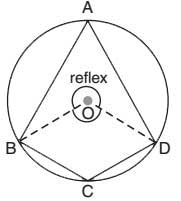Proof: